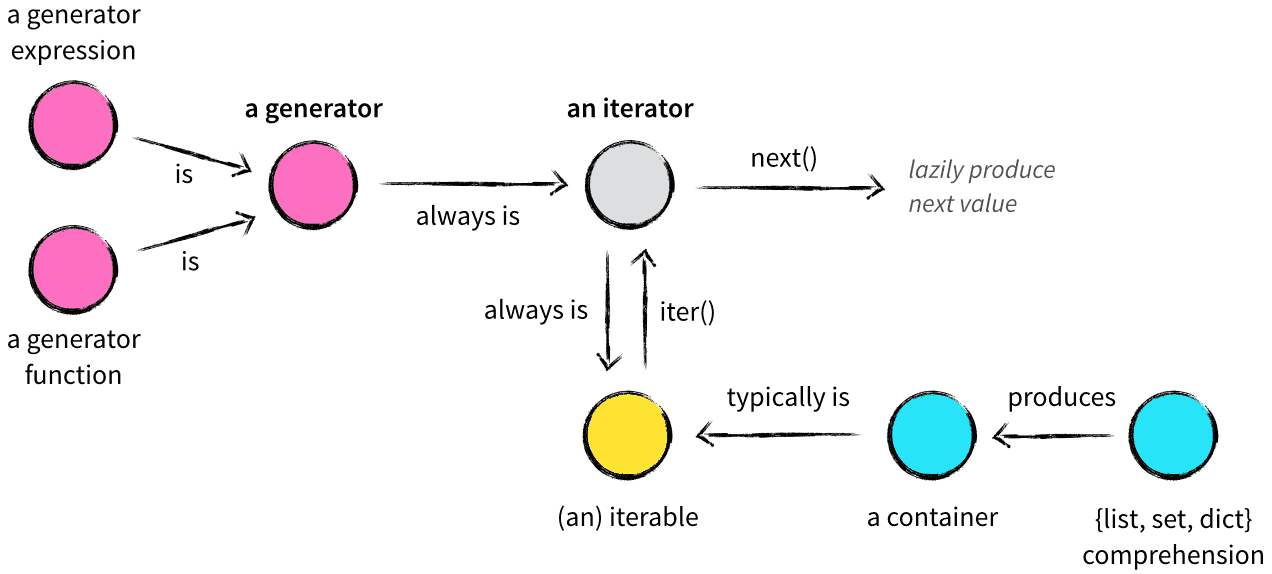# 发电机和发电机,生成器,生成式

## 1.生成器

• 延迟计算、惰性求值
• 节省内存，高效
• 缺点：无法随机存取

### 1.1yield表达式

``````#通过函数和yield关键字生成
#使用了 yield 的函数被称为生成器（generator）
#yield语句一次返回一个结果，在每个结果中间，挂起函数的状态，以便下次从它离开的地方继续执行
def test(n):
for i in range(1,n + 1):
yield i
#print(i)
#得到生成器
result = test(10)
print(result)
#可以使用next函数获取下一个值
print(next(result))

#生成器只能遍历一次, 所以下面不会有任何输出
for x in result:
print(x)

#可以这样遍历
for x in test(10):
print(x)
``````

### 1.2生成器表达式

（表达式 for var in 可迭代对象）

``````#将列表生成式中的[]替换成()
ge = (x for x in range(1,6))
print(ge,type(ge))

#生成器需要通过next()方法获取数据，调用一次则返回一个数据
print(next(ge))
print(next(ge))
print(next(ge))
print(next(ge))
print(next(ge))

#注意：如果通过next函数获取生成器中的数据，当数据取完之后，
#将不能再调用next函数，否则出现StopIteration
#print(next(ge))  #StopIteration

#生成器主要通过for-in的方式进行遍历
for x in ge:
print(x,end=' ')
``````

## 2.迭代器### 2.1 迭代对象

• 数据结构：list、set、tuple、dict、string
• generator【生成器】【（），函数结合yield】
``````#引入 from collection  import Iterable
from collections import Iterable
print(isinstance("",Iterable))#True
print(isinstance({},Iterable))#True
print(isinstance((),Iterable))#True
print(isinstance(1,Iterable))#False
``````

### 2.2 迭代器

``````from collections import  Iterator,Iterable
print(isinstance([],Iterator))  #False
print(isinstance((),Iterator))  #False
print(isinstance({},Iterator))  #False
print(isinstance("",Iterator))  #False
print(isinstance((x for x in range(0,6)),Iterator))  #True 生成器是迭代器
print(isinstance((x for x in range(0,6)),Iterable)) #True 生成器也是迭代对象
``````

### 2.3 将迭代对象转换为迭代器

iter():将可迭代对象转化为迭代器【主要针对list、set、tuple、dict、string】

``````print(isinstance(iter([]),Iterator)) #True
print(isinstance(iter(()),Iterator)) #True
print(isinstance(iter({}),Iterator)) #True
print(isinstance(iter(""),Iterator)) #True

l2 = iter(l1)  #将列表转换为迭代器
print(next(l2))  #使用next获取迭代器中的元素

while True:
try:
print(next(l2))  #可能出问题的代码放到try块中
except StopIteration: #捕获异常
break  #终止循环
``````Python Free

QQ：417803890